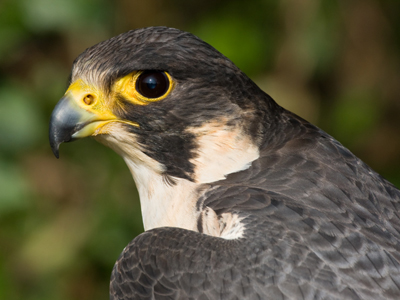A Peregrine Falcon can fly at 90km/h.

# Speed 2

This Science quiz is called 'Speed 2' and it has been written by teachers to help you if you are studying the subject at middle school. Playing educational quizzes is a fabulous way to learn if you are in the 6th, 7th or 8th grade - aged 11 to 14.

It costs only \$12.50 per month to play this quiz and over 3,500 others that help you with your school work. You can subscribe on the page at Join Us

Speed can also be thought of as the rate at which something moves. Speed is an important subject in the field of Science. The following questions are about calculating distance. Distance = speed x time.

See how fast you can do our second quiz in the series all about speed!

1.
A car travels at 50mph for 2 hours. How far does it go?
10 miles
50 miles
100 miles
100 kilometers
Distance = 50mph x 2h = 100 miles
2.
A cyclist has a speed of 10km/h. She cycles for 2 hours - how far does she go?
5 kilometers
12 kilometers
20 kilometers
25 kilometers
Distance = 10km/h x 2h = 20 kilometers
3.
A dog runs at 15m/s for 30 seconds. How far does he go?
45m
450m
2km
4.5km
15m/s x 30s = 450m
4.
An athlete runs at 8m/s for 1 minute. How far do they go?
48m
80m
480m
4.8km
1 minute = 60 seconds so distance = 8m/s x 60s = 480m
5.
If time is measured in hours, speed in km/h, distance .......
is in centimeters
is in meters
is in kilometers
is in miles
6.
If time is measured in hours, speed in mph, distance .......
is in centimeters
is in meters
is in kilometers
is in miles
7.
A car travels at 40mph for 60 minutes. How far does it go?
40 kilometers
40 miles
60 miles
240 miles
60 minutes = 1hour so distance = 40mph x 1h = 40 miles
8.
If time is measured in seconds, speed in m/s, distance .......
is in centimeters
is in meters
is in kilometers
is in miles
9.
A peregrine falcon can fly at 90km/h. How far could it fly in 20 minutes?
4.5 kilometers
18 kilometers
30 kilometers
1,800 kilometers
20 minutes = 20/60 = 1/3 hour so distance = 1/3h x 90km/h = 30km
10.
A cyclist has a speed of 10km/h. She cycles for 120 minutes - how far does she go?
10 kilometers
12 kilometers
20 kilometers
1,200 kilometers
120 minutes = 2 hours so distance = 10km/h x 2h = 20km
Author:  Sue Davison